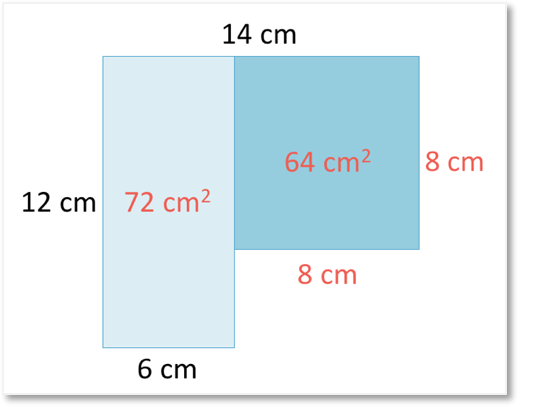# How to Find the Volume of a Cuboid

How to Find the Volume of a Cuboid• A cuboid is also known as a rectangular prism and it is shaped liked a box.
• A cuboid is a 3D shape, which means that it has height, length and width.
• The volume of a cuboid can be measured by how many 1 cm3 cubes fit inside it.
• These two cuboid examples have a top area of 4 x 3 = 12 cm2.
• Therefore there are 12 cubes in each layer of these cuboids.
• The volume of the cuboid with one layer is 12 cm3.
• The volume of the cuboid with two layers is 24 cm3.
• To find the volume, we multiply the length x width x height for each cuboid.
• Our for volume, cm3, contain a three because we multiplied in three directions.
The volume of a cuboid is found by multiplying the length x width x height.

The units are cubed, e.g. ‘cm3‘.• The volume of this cuboid is found by by multiplying the length x width x height in any order.
• 4 cm x 3 cm x 3 cm = 36 cm3.
• The volume of the cuboid is 36 cm3.
• The volume of this cuboid tells us how many 1 cm3 cubes fit inside it.
• We can count these cubes by first finding the number of cubes in each layer.
• The number of cubes in each layer is the length x width of the cuboid.
• 4 x 3 = 12 so there are 12 cubes in each layer.
• There are 3 layers in total, so three layers of 12 cubes.
• 12 x 3 = 36 so there are 36 cubes inside the cuboid.Supporting Lessons# Finding the Volume of a Cuboid

## How to Find the Volume of a Cuboid

To find the volume of a cuboid, multiply its length, width and height together in any order. The units of the length, width and height need to be the same and this unit is cubed in the final volume answer.

For example, a cuboid with a length of 5 cm, a width of 2 cm and a height of 3 cm has a volume of 5 x 2 x 3 = 30 cm3.It does not matter in which order the numbers are multiplied together. 5 x 2 x 3 and 2 x 3 x 5 both equal 30.

The length, width and height of a cuboid must be right angles to each other. When finding the volume of a cuboid, it is important to make sure that the 3 dimensions of length, width and height are all at right angles to each other when measuring them. It is possible to make the mistake of reading the wrong dimension and read one of the dimensions twice.

The height of a cuboid is always measured as the vertical distance from the bottom to the top. In this example, the height is 3.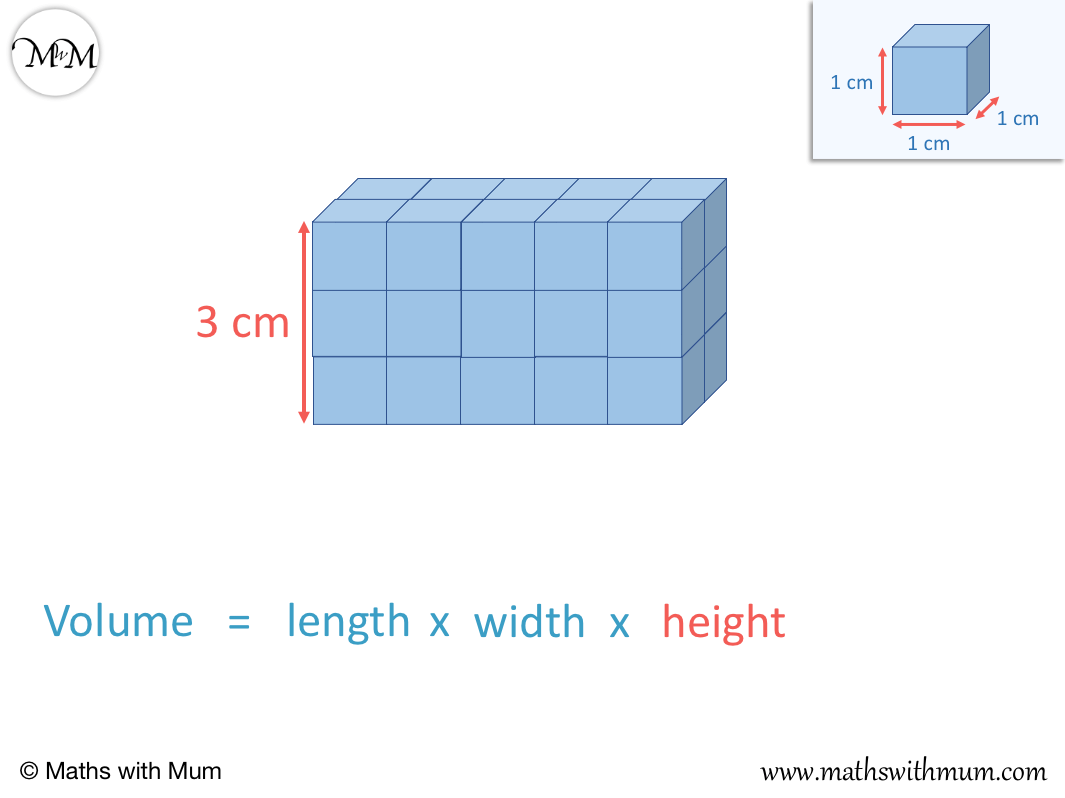Aside from the height, there are two remaining sides. The length is the longest side and the width is the shortest side.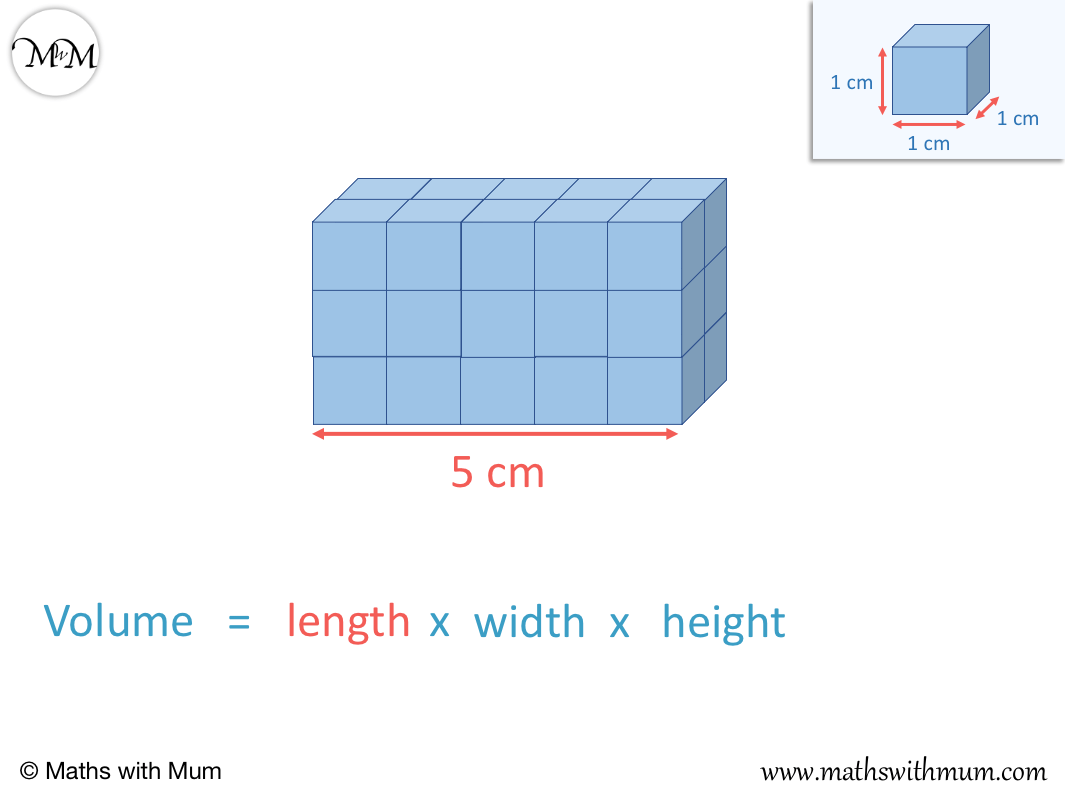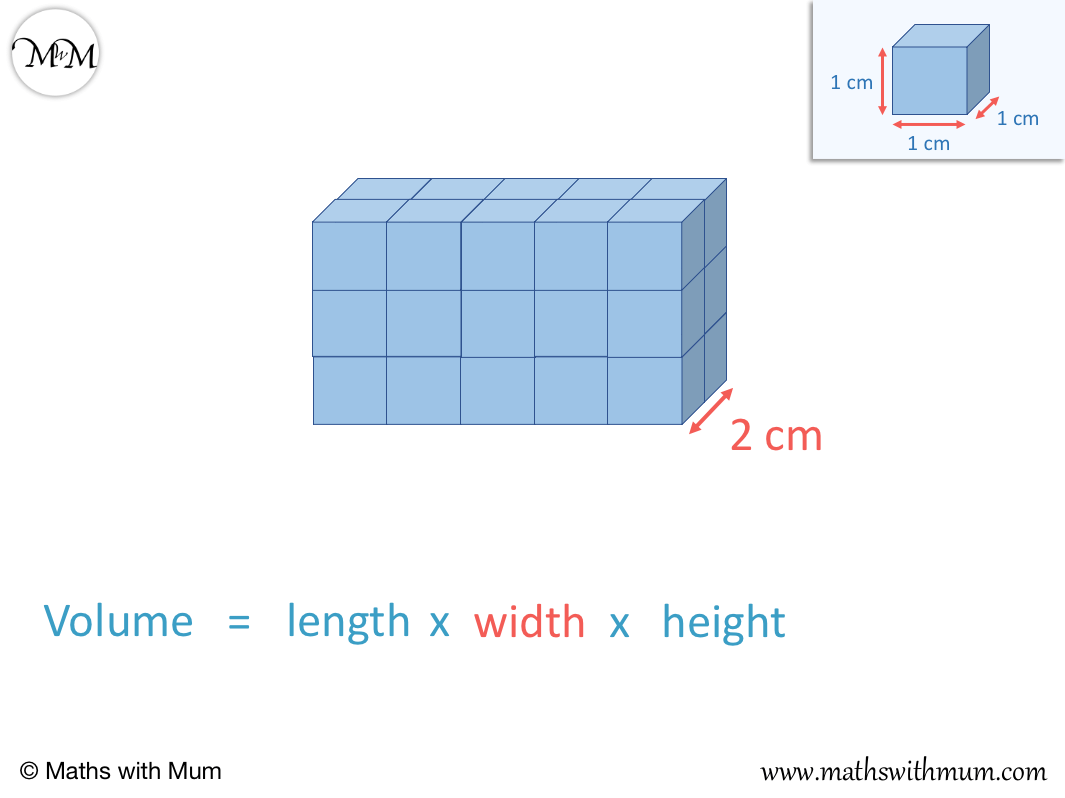The length of this cuboid is 5 and the width is 2.

## The Formula for the Volume of a Cuboid

The formula for the volume of a cuboid is V = l x w x h, where l is the length, w is the width and h is the height. This formula can be written more concisely as V = lwh. The volume is in cubic units.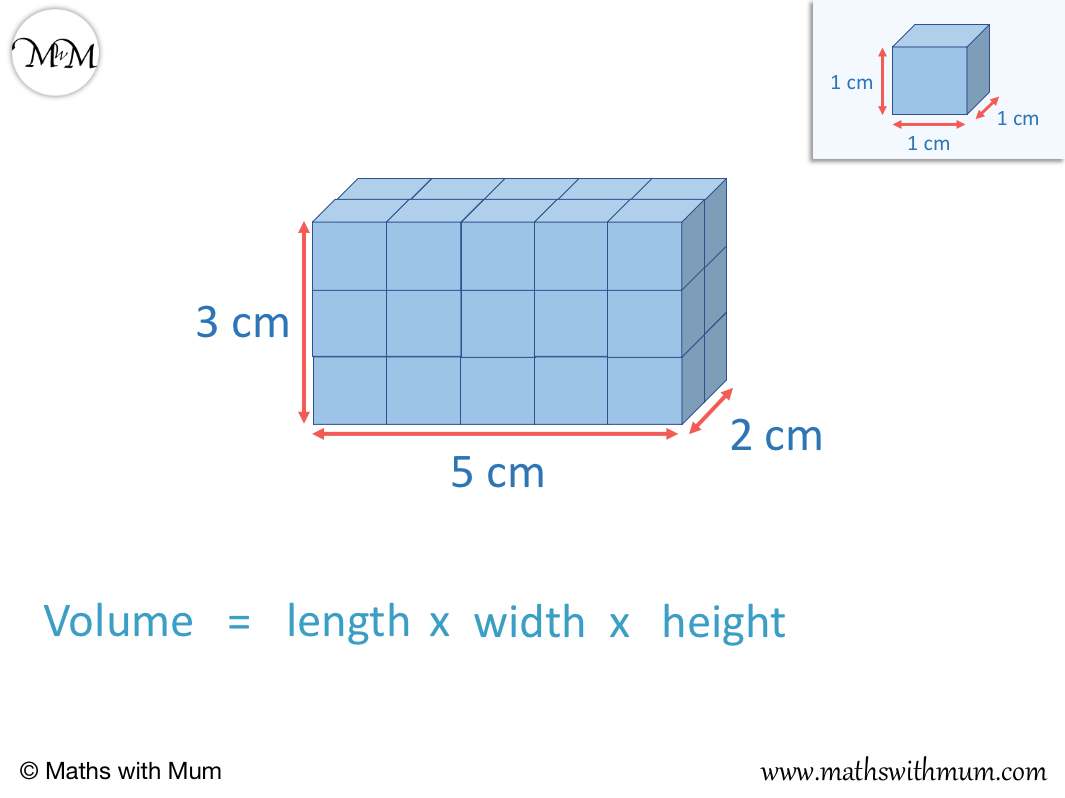The volume of a cuboid is length x width x height. This equation can be simplified into a formula by replacing length with the letter l, width with w and height with h.

V = l x w x hWe don’t tend to write multiplication signs when using algebra. Writing the formula V = l x w x h without multiplication signs allows us to write the formula for a volume of a cuboid in its simplest form as V = lwh.

A cuboid is also known as a rectangular prism. Therefore finding the volume of a rectangular prism is the exact same method used for finding the volume of a cuboid.

To find the volume of a rectangular prism, multiply the length, width and height together in any order. For example, a rectangular prism with a length of 4 cm, a width of 3 cm and a height of 2 cm has a volume of 4 x 3 x 2 = 24 cm3.The formula for the volume of a rectangular prism is V = l x w x h.

## Volume of a Cuboid by Counting Cubes

The volume of a cuboid can be found by counting how many centimetre cubes its contains. It is easiest to count the cubes in one layer and then to multiply this by the number of layers in the cuboid.

A cuboid has three dimensions. The dimensions are the height, length and width.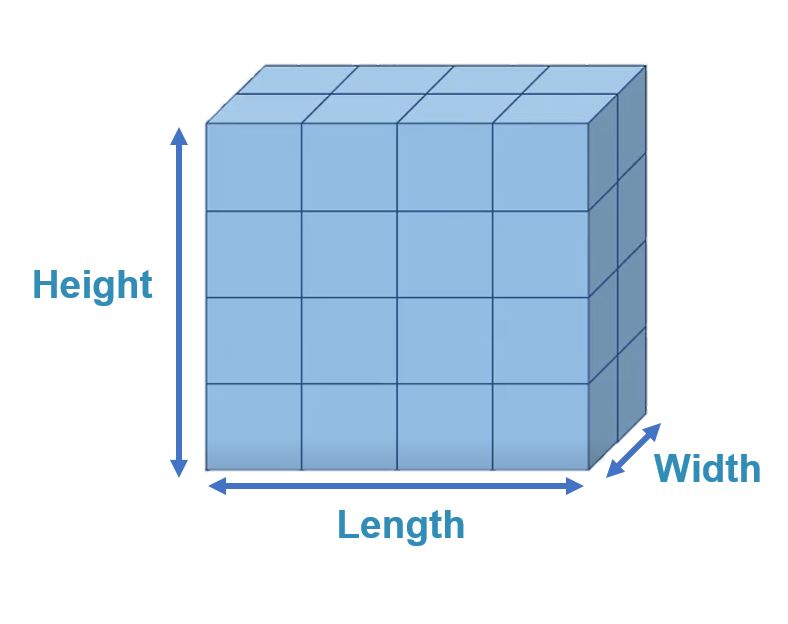Here is a cuboid with only one layer.

There are twelve cubes in total. We know this because the length is 4 and the width is 3. To find the number of cubes in each layer of a cuboid, multiply the length by the width. 4 x 3 = 12 and so, there are 12 cubes in this layer.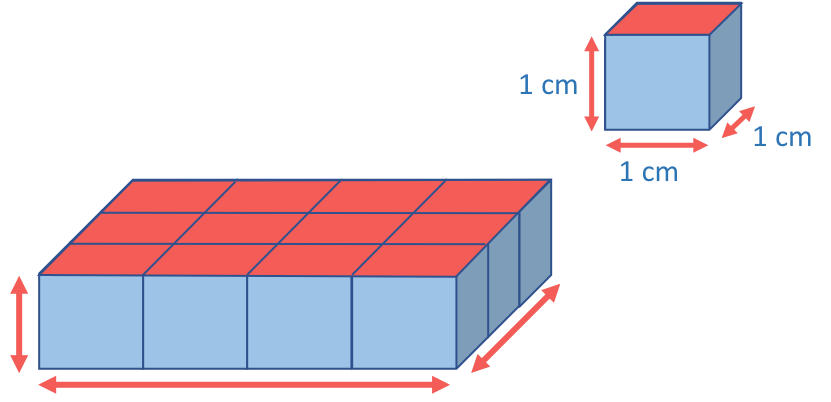The volume of this cuboid is 12 cubic centimetres, 12 cm3.

When teaching volume by counting cubes, it is useful to have some physical cubes on hand to make the shapes with. The number of cubes can easily be counted as they are added.

Here is another cuboid, which is the same as before but has an extra layer.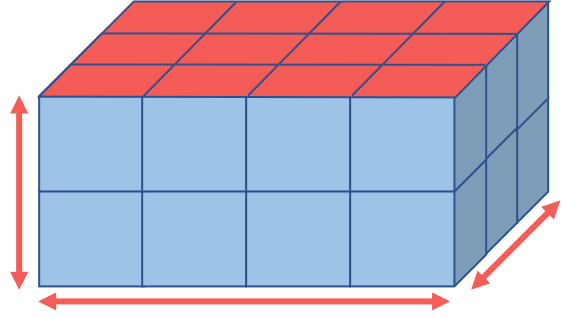The number of cubes in each layer has not changed. The length is still 4, the width is still 3 and so, the number of cubes in each layer is 4 x 3 = 12.

However we now have two layers of 12. 2 lots of 12 is found by multiplying 2 and 12. 2 x 12 = 24.

The volume of this cuboid is 24 cm3, 24 cubic centimetres.

Here is a cuboid with 3 layers. There are still 12 cubes in each layer.We have 3 layers of 12 cubes. 3 x 12 = 36 and so the volume of this cuboid is 36 cm3, 36 centimetres cubed.

The number of cubes in each layer can be seen by looking at the cuboid directly from the top.The volume of a cuboid is given by length x width x height. The length x width tells us the number of cubes in each layer of the cuboid and the heigth tells us how many layers there are. Multiplying the three dimensions together tells us the total number of centimetre cubes and hence, the volume in cubic centimetres.

## Units of Volume for a Cuboid

The length of each side of a cuboid can be measured in centimetres, metres or any other unit of length. The units of measurement for volume must be this unit of length cubed.

For example, if the side of a cuboid is measured in centimetres, then the volume is measured in centimetres cubed (cm3).

If the side of a cuboid is measured in metres, then the volume is measured in metres cubed, (m3).

The small three in the unit of measurement is pronounced as ‘cubed’. This number tells us how many dimensions (directions) have been multiplied together.

For example, here is a rectangle with a length of 4 cm and a width of 3 cm. Its area is found by multiplying the length and width. 4 cm x 3 cm = 12 cm2.In the calculation of 4 cm x 3 cm = 12cm2, we multiply a centimetre by a centimetre. To show that two centimetres lengths are multiplied together, we write 12 cm2. The 2 shows us that 2 lengths were multiplied to get this result.

If we have one row of twelve 3D cubes, then we have a volume of 12 cm3.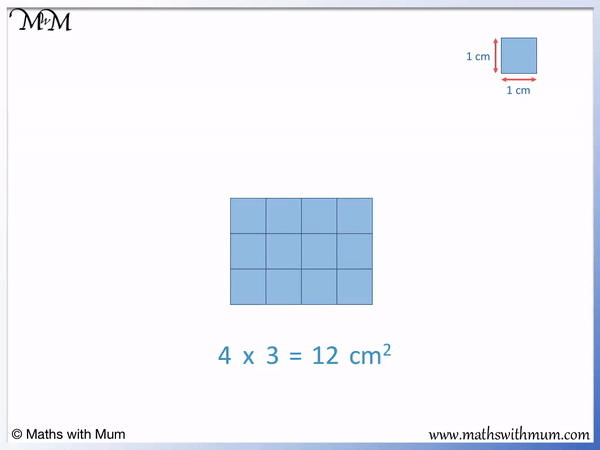This shape is now 3D. A 3 dimensional shape can be physically held as it has length, width and height. There is only one layer of cubes, so this shape has a height of 1.

The volume is found by 4 cm x 3 cm x 1 cm = 12 cm3.

When finding the volume, three sides measured in centimetres are multiplied together and so, the units of volume are cm3.

Here is a cuboid with three layers of 12.

The volume is 4 cm x 3 cm x 3 cm = 36 cm3.Again, we multiplied the length, width and height together. Multiplying in 3 directions means that a three is needed as the power of our units.

cm x cm x cm = cm3.

cm3 is pronounced as centimetres cubed or cubic centimetres.Now try our lesson on Area of a Compound Shape where we learn find the area inside a shape made up of more than one rectangle.